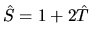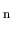## T-matrix method (EBCM)

The T-matrix or transition matrix is well known in the quantum theory of scattering  where one also uses the Heisenberg S-matrix connected with the T-matrix by the relation. In contrast to the coordinate representation in the integral equation method, where the scattered and incident fields are connected by the Green function, the T-matrix connects the coefficients of the expansions of the scattered and incident fields in terms of a complete system of the vector basis functions. In the theory of light scattering the T-matrix first appeared in the pioneer works of P.C. Waterman in 1965-1979 [496,497,498,499]. The initial formulation used the Huygens principle for perfectly conducting  and dielectric  particles was generalized in  where also different ways of formulation of the method equations without the Huygens principle and fictive sources were considered. Although the papers of Waterman [496,497,498,499] contained exhaustive information on principal and technical features of the method, it attracted the attention of scientists in the optics only after the publication of the paper of P.W. Barber and C. Yeh in the Applied Optics in 1975  where the method was formulated using the Shelkunov equivalence theorems and called the Extended Boundary Condition Method - EBCM. Now both names are nearly equally used. The further works of Barber's group [142,143,144,145,146,227,324,328,493,494,508] also had a noticeable influence on the growth of the method popularity. The generalization of the T-matrix method for multi-particle configurations were developed in [300,395,464], for multi-layered scatterers in [300,396,493], and chiral particles of non-spherical shape in [314,319]. For strongly aspherical weakly absorbing particles, the convergence of the T-matrix method is rather weak, therefore an iterative modification of the algorithm was suggested in  with further development and applications in [266,268,269,316,318]. From the computational point of view, the method convergence problems for strongly aspherical particles of large size are connected with the errors of calculations of the integrals defining the T-matrix [49,60], therefore a simple and effective way of solution of this problem is the transition to representation of numbers in the computer with extended accuracy [359,361].

The analysis of the scattering characteristics of polydisperse ensembles of particles with random orientation needs two additional integrations - over size and orientation of scatterers and hence larger computational resources. Therefore, very important is the derivation of equations for the analytic orientational averaging of all physically interesting optical characteristics: the integral cross-sections and Mueller matrix [54,98,281,345,350,348], the extinction matrix of the transfer equation [344,348] and small-angle scattering flux [57,388]. Using the apparatus of the quantum theory of angular momentum we demonstrated that the equations for the orientational averaging of the T-matrix and its bilinear forms (and hence the physically observer quantities) do not depend on the particle nature and scattering waves [98,281]. In the works of M.I. Mishchenko  and L.E. Paramonov [59,60] (see also the references in ) this approach was generalized for disperse systems with ordered orientation of particles. The numerical algorithms built on the equations of the analytical orientational averaging were found to be the most efficient ones for the analysis of light scattering characteristics of ensembles of non-spherical particles with random orientation (see the works of Mishchenko's group at the NASA Goddard Institute for Space Studies [345,346,347,348,349,350,351,352,353,354,355,356,357,358,359,360,361,362] and also the papers [47,48,49,60,63,64,78,102,103] in Russian).

The important advantage of the T-matrix method is its natural adaptation to the problems of the multi-scattering wave theory [331,468,469,511,471,480,481,482,485,486,487], radiative transfer equations [60,472,473], scattering by cluster of particles [195,353,354,355,362], particles of irregular shape  and Chebyshev T-particles , Raman scattering , extinction by polydisperse and polymorphic metal zoles [102,103,282,283] and so on. Thus, the T-matrix approach is practically universal for all application fields of the light scattering theory. Concluding the discussion of this method, we insistently refer a reader to the reviews [362,483] where the exhausted literature on the theory and applications of the T-matrix method is collected.To the top
Created by V.I.